По-русски

# Symbol and Definition

 μi A.C. Initial Permeability μi is defined as the limited value of a ferrite core at the origin of the curve of initial magnetization: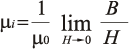μ0 : Permeability of vacuum B: A.C.magnetic flux density H: A.C.magnetic field strength μa Amplitude Permeability similar with i, but magnetized by a large amplitude sine field. Tanδ/μi Relative Loss Factorloss at low induction level. PV Power lossloss at high flux density level. Bms Effective Saturation Magnetic Flux Density (mT) Brms Residual Magnetic Flux Density (mT) Hc Coercive Force (Oersteds) (A/m) αF Temperature Factor of Permeability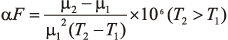μ1 : Permeability of T1 μ2 : Permeability of T2 ηB Hysteresis Material Constant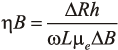ΔRh: hyseresis loss resistance ω: angular frequency L : inductace of coil with the core μe : effectve permeability ΔB: amplitude magnetc flux of density DF Disaccommodation Factor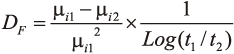μi1 : permeability measured at time t1 after demagnetization μi2 : permeability measured at time t2 after demagnetization
 Tc Curie Temperature temperature at which a ferrite loses is ferromagnetism Specific Resistivity (m) d Apparent density, The Apparent density is defined as a weigh per unit volume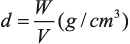where W: weight of the magnetic core(g) V: volume of the magnetic core(cm3) AL(nH) Inductance Factor Inductance of a coil on a specified core divided by the square of the number of turns.(Unless otherwise specified the inductance test conditions for the inductance factor are at flux density<10 gauss). Inductance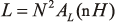Effective Core Parameters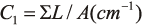The summation of the magnetic path lengths of each section of a magnetic circuit divided by the corresponding magnetic area of the same section.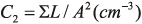The summation of the magnetic path lengths of each section of a magnetic circuit divided by the square of the corresponding magnetic area of the same section. Le=C1²/C2(cm) Effective magnetic path length Ae=C1/C2(cm²) Effective cross-sectional area Ve=C1³/C2²(cm³) Effective core volume C1 (mm-1) Core constant Aw (mm²) Winding area of core Ac (mm²) cross-sectional centre leg area W (g) Approx.weigh of core

2011-09-30 18:13:43